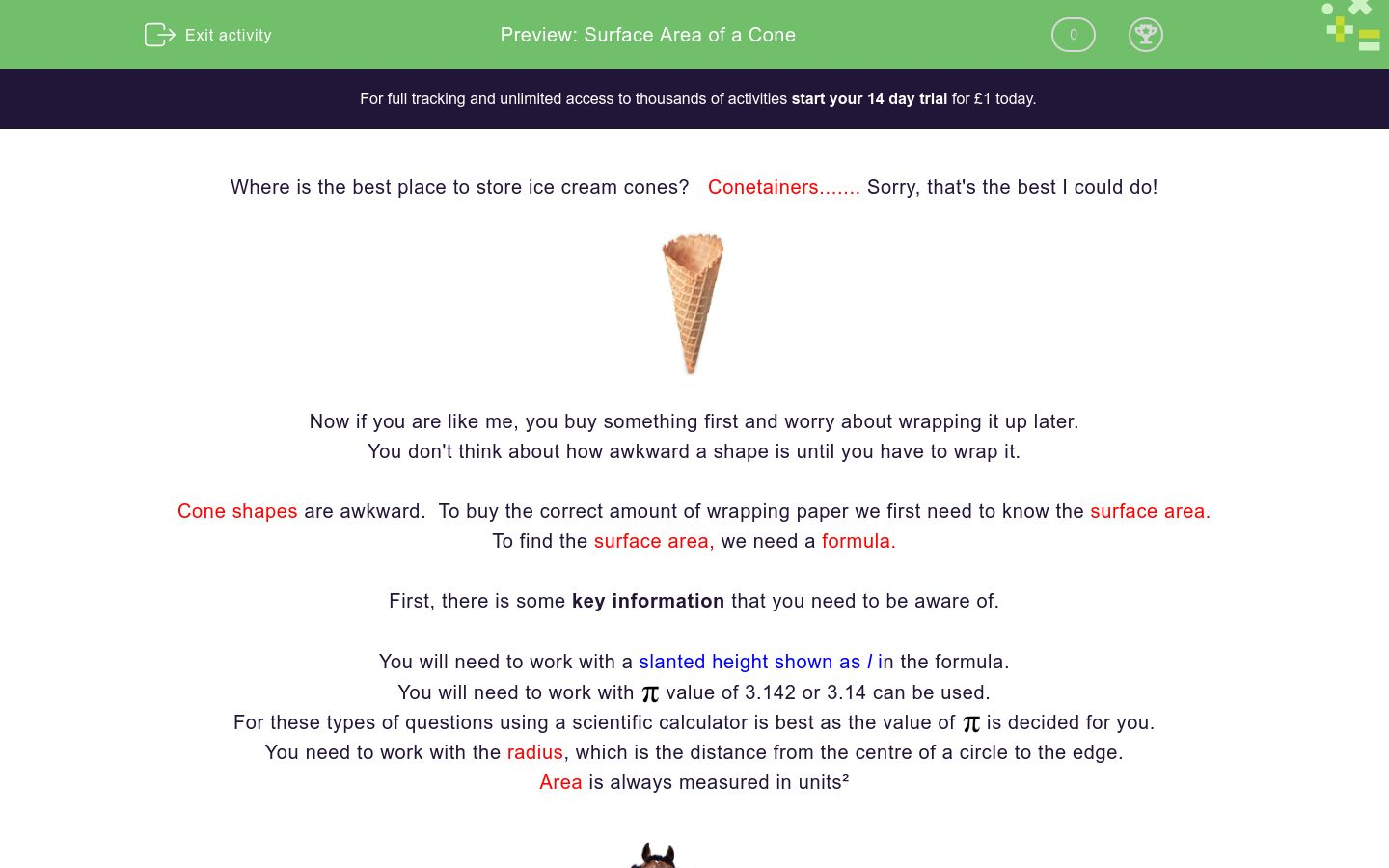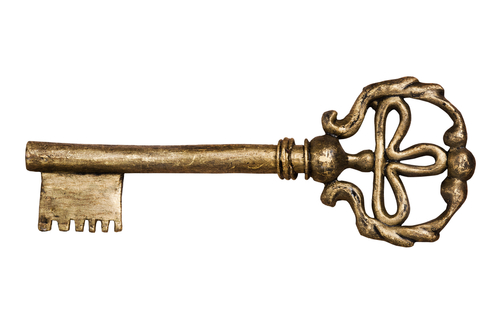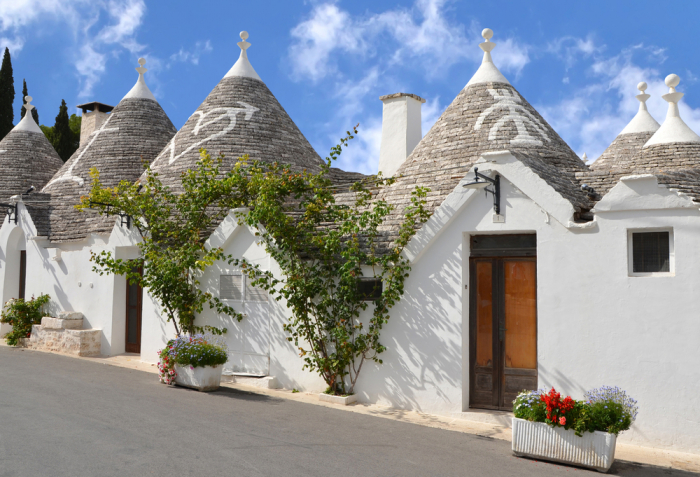# Surface Area of a Cone

In this worksheet, students will learn the formula for finding the surface area of a cone and be able to apply it.Key stage:  KS 4

GCSE Subjects:   Maths

GCSE Boards:   AQA, Eduqas, Pearson Edexcel, OCR

Curriculum topic:   Geometry and Measures, Mensuration

Curriculum subtopic:   Mensuration and Calculation, Volume and Surface Area Calculations

Difficulty level:### QUESTION 1 of 10

Where is the best place to store ice cream cones?   Conetainers....... Sorry that is the best I could do.Now if you are like me, you buy something first and worry about wrapping it up later.

You don't think about how awkward a shape is until you have to wrap it.

Cone shapes are awkward.  To buy the correct amount of wrapping paper we first need to know the surface area.

To find the surface area, we need a formula.First there is some key information that you need to be aware of.

You will need to work with a slanted height shown as in the formula.

You will need to work withvalue of 3.142 or 3.14 can be used.

For these types of questions using a scientific calculator is best as the value ofis decided for you.

You need to work with the radius, which is the distance from the centre of a circle to the edge.

Area is always measured in units²Nay bother.

The formula comes in three stages

1.  To find the area of the curved face the formula isx r x l

2. To find the area of the base the formula isx r²x r x lx r²

It can be a bother having to learn so many formulas, but let us look at this closely.

A cone has a circular shape so the formula for finding an area of a circle is in it.x r²

This gives us the area of the surface of the base.

The other part of the formula starts the same, uses the radius, so the only other thing to think about is selecting (l) the slant height.

Question setters often give a vertical  and a slant height, so beware.  Think that a cone slants. That is how I rememberLet's get started, I bet you can't conetain yourself.Find the surface area  of this cone.  The radius is 4 cm. The slant height is 7 cm.

Part 1x r x lx 4 x 7 = 87.96 cm²

Part 2x r x rx 4 x 4 = 50.27 cm²

Part 3

87.96 + 50.27 = 138.23 cm²

Just beware of a few things to look out for.

Sometimes you are given the diameter of the cone, remember to halve it to get the radius.Select the two parts that make the formula that you need to find the surface area of a cone.

Π x r x l

Π x r

Π x r x h

Π x r x r

Π x r² x l

Π x r x r x lWhat is the surface area of this cone with a radius of 5 cm, height  11 cm and a  slant height of 9 cm?Cone shaped Christmas trees are all the rage these days.

What is the surface area of this one? The radius is 8 cm and the slant height of 12 cm.

Match up the following

## Column B

Surface area of a cone, radius 6 cm, slant height...
141.37 cm³
Surface area of a cone, radius 4 cm, slant height ...
264.00 cm²
Surface area of a cone, radius 7 cm, slant height ...
115.45 cm³
Surface area of a cone, radius 3.5 cm, slant heigh...
241.90 cm³Calculate the surface area of this cone.

It has a diameter of 14 cm and a  slant height of 5 cm.In the Puglia area of Italy, there are traditional houses called trulli houses.  There roofs are shaped like cones.

Answers have been rounded to the nearest whole number.

 172.79 m² 486.95 m² 65.97 m² 157 m² 351.58 cm² 139 m² 279 m² 241.95 m² 113.00 m² Surface area with a radius of 3 m and a slant height of 4 m Surface area with a radius of 4 m and a slant height of 5 m Surface area with a radius of 5 m and a slant height of 6 m Total surface areaWhat is the surface area of this cone?

Slant Height 1/Π diameter 8 cm.

48.91 cm ³

42.35 cm ³

54.27 cm ³

55.68 cm ³Find the surface area of this cone.  The radius is 6 cm and the slant height is a third more than the radius.What is the surface area of this cone? Radius 3 cm, slant height 5 cm.

57.23 cm²

74.21 cm²

75.40 cm²

47.12 cm²Calculate the surface area of this cone?

R - 4 cm, h = 6 cm, l = 5 cm

113.10 cm

121.09 cm

115.20 cm

142.61 cm

• Question 1Select the two parts that make the formula that you need to find the surface area of a cone.

Π x r x l
Π x r x r
EDDIE SAYS
Did you remember the area of a circle Π x r², This give us one part of the formula. Did you remember that the slant height is shown by l in formulas? Like anything once you get used to working with the formula, you will remember it better.
• Question 2What is the surface area of this cone with a radius of 5 cm, height  11 cm and a  slant height of 9 cm?

219.91
EDDIE SAYS
Part 1 Π x 5 x 9 =141.37 cm² Part 2 Π x r x r = 78.54 cm² Part 3 141.37 + 78.54 = 219.91 cm²
• Question 3Cone shaped Christmas trees are all the rage these days.

What is the surface area of this one? The radius is 8 cm and the slant height of 12 cm.

EDDIE SAYS
Do you try and find your Christmas presents before the big day? Just think of the formula as a gift. Π x 8 x 12 = 301.59 cm² Π x 8 x 8 = 201.06 cm² 301.59 + 201.06 = 502.65 cm² I don't think I would fancy wrapping that.
• Question 4

Match up the following

## Column B

Surface area of a cone, radius 6 ...
264.00 cm²
Surface area of a cone, radius 4 ...
141.37 cm³
Surface area of a cone, radius 7 ...
241.90 cm³
Surface area of a cone, radius 3....
115.45 cm³
EDDIE SAYS
Is this getting easier now? I wish my wrapping of awkward shapes was. Practice makes perfect This is all there is to it. Honestly
• Question 5Calculate the surface area of this cone.

It has a diameter of 14 cm and a  slant height of 5 cm.

263.89
EDDIE SAYS
Did you spot it? You were given the diameter so needed to halve it first to get the radius. π x 7 x 5 then π x 7² Now just add together.
• Question 6In the Puglia area of Italy, there are traditional houses called trulli houses.  There roofs are shaped like cones.

Answers have been rounded to the nearest whole number.

 172.79 m² 486.95 m² 65.97 m² 157 m² 351.58 cm² 139 m² 279 m² 241.95 m² 113.00 m² Surface area with a radius of 3 m and a slant height of 4 m Surface area with a radius of 4 m and a slant height of 5 m Surface area with a radius of 5 m and a slant height of 6 m Total surface area
EDDIE SAYS
Hopefully calculating the surface area is second nature to you now. The added extra is knowing that total is looking for an addition at the end. I wonder what they keep in the attic of a roof shaped like this.
• Question 7What is the surface area of this cone?

Slant Height 1/Π diameter 8 cm.

54.27 cm ³
EDDIE SAYS
Don't let a number that looks awkward put you off. Put this into your calculator as normal. This is the beauty of having a scientific calculator. π x 4 x 1 ÷ π + π x 4 x 4 Did your eagle eye spot that you were given the diameter. What do we have to do to get the radius? of course halve the diameter.
• Question 8Find the surface area of this cone.  The radius is 6 cm and the slant height is a third more than the radius.

121.47
EDDIE SAYS
Just to keep you on your toes. First you need to add a third more to the radius. 1/3 of six is 2 so slant height is 8 cm. Situation normal. π x r x l + π x r x r
• Question 9What is the surface area of this cone? Radius 3 cm, slant height 5 cm.

47.12 cm²
EDDIE SAYS
Ah, did you spot what I did there. The ice cream cone doesn't have a fixed base so you only needed the first part of the formula. π x r x l Forgive me, at least you can cram as much ice cream into the cone as you want.
• Question 10Calculate the surface area of this cone?

R - 4 cm, h = 6 cm, l = 5 cm

113.10 cm
EDDIE SAYS
π x 4 x 5 + π x 4 x 4 = 113.10 cm² (2 d.p) Did you pick the correct height i.e the slant height represented by the letter l. That is the important bit to remember. Think that a cone slants at the surface, so that is a good way to remember which height to use.
---- OR ----

Sign up for a £1 trial so you can track and measure your child's progress on this activity.

### What is EdPlace?

We're your National Curriculum aligned online education content provider helping each child succeed in English, maths and science from year 1 to GCSE. With an EdPlace account you’ll be able to track and measure progress, helping each child achieve their best. We build confidence and attainment by personalising each child’s learning at a level that suits them.

Get started